## Regression Numerical Example

Suppose we have the following 5 data points and we want to predict the population data for the year 2005 using linear regression model. In this section, we will use hand calculation or spreadsheet using linear regression formula. You may download the spreadsheet example of this tutorial hereThe formula for linear regression is given below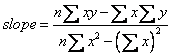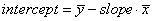To use that formula, we make a table consists of four columns. The fist two columns are the data Year as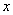and Population as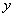. The third column is multiplication of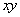for each row and the last column is square of. You can use MS Excel spreadsheet to ease your hand computation.

 x y xy x^2 Year Population square error sq. mean difference 1980 2.1 4158 3920400 0.0004 1.80 1985 2.9 5756.5 3940225 0.0196 0.29 1990 3.2 6368 3960100 0.0576 0.06 1995 4.1 8179.5 3980025 0.0004 0.44 2000 4.9 9800 4000000 0.01 2.13 sum 9950 17.2 34262 19800750 0.088 4.71 average 1990 3.44 count n = 5

We sum all the five rows and for the first two columns, we calculate the average of year as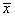=1990 and the average of population as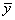= 3.44. Since we have 5 data, then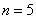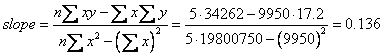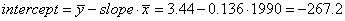Thus, we get the regression line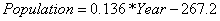Using this regression line, we can predict the number of population in the city for year 2005. By inputting 2005 to the year we get population = 5.48 (x hundred thousand persons).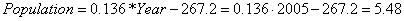The R-squared value can be computed as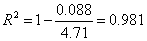Preferable reference for this tutorial is

Teknomo, Kardi (2015) Regression Model using Microsoft Excel. http://people.revoledu.com/kardi/tutorial/Regression/